Related Articles
Rotate axis tick labels in Seaborn and Matplotlib
• Last Updated : 25 Feb, 2021

Seaborn and Matplotlib both are commonly used libraries for data visualization in Python.  We can draw various types of plots using Matplotlib like scatter, line, bar, histogram, and many more. On the other hand, Seaborn provides a variety of visualization patterns. It uses easy syntax and has easily interesting default themes. It specializes in statistics visualization.

Creating a basic plot in Matplotlib

## Python3

 `import` `numpy as np``import` `matplotlib.pyplot as plt`` ` `data ``=` `{``'Cristopher'``: ``20``, ``'Agara'``: ``15``, ``'Jayson'``: ``30``,``        ``'Peter'``: ``35``}``names ``=` `list``(data.keys())``age ``=` `list``(data.values())`` ` `fig ``=` `plt.figure(figsize``=``(``10``, ``5``))`` ` `# creating the bar plot``plt.bar(names, age, color``=``'blue'``, width``=``0.4``)`` ` `plt.xlabel(``"Names"``)``plt.ylabel(``"Age of the person"``)``plt.show()`

Output: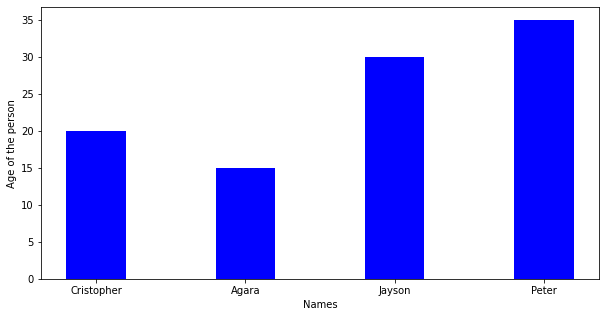Creating basic plot in Seaborn

## Python3

 `import` `seaborn as sns``import` `matplotlib.pyplot as plt`` ` `sns.barplot(x``=``[``"Asia"``, ``"Africa"``, ``"Antartica"``, ``"Europe"``],``            ``y``=``[``90``, ``60``, ``30``, ``10``])`` ` `plt.show()`

Output:While plotting these plots one problem arises -the overlapping of x labels or y labels which causes difficulty to read what is on x-label and what is on y-label. So we solve this problem by Rotating x-axis labels or y-axis labels.

Rotating X-axis Labels in Matplotlib

We use plt.xticks(rotation=#) where # can be any angle by which we want to rotate the x labels

## Python3

 `import` `numpy as np``import` `matplotlib.pyplot as plt`` ` `data ``=` `{``'Cristopher'``: ``20``, ``'Agara'``: ``15``, ``'Jayson'``: ``30``,``        ``'Peter'``: ``35``}``names ``=` `list``(data.keys())``age ``=` `list``(data.values())`` ` `fig ``=` `plt.figure(figsize``=``(``10``, ``5``))`` ` `# creating the bar plot``plt.bar(names, age, color``=``'blue'``, width``=``0.4``)`` ` `plt.xlabel(``"Names"``)``plt.xticks(rotation``=``45``)``plt.ylabel(``"Age of the person"``)``plt.show()`

Output: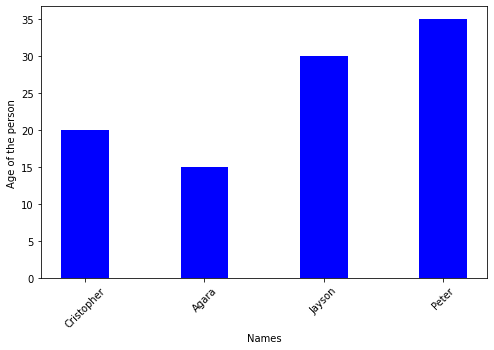Rotating X-axis Labels in Seaborn

By using FacetGrid we assign barplot to variable ‘g’ and then we call the function set_xticklabels(labels=#list of labels on x-axis, rotation=*) where * can be any angle by which we want to rotate the x labels

## Python3

 `import` `seaborn as sns``import` `matplotlib.pyplot as plt`` ` `g ``=` `sns.barplot(x``=``[``"Asia"``, ``"Africa"``, ``"Antartica"``, ``"Europe"``],``                ``y``=``[``90``, ``30``, ``60``, ``10``])``g.set_xticklabels(``    ``labels``=``[``"Asia"``, ``"Africa"``, ``"Antartica"``, ``"Europe"``], rotation``=``30``)``# Show the plot``plt.show()`

Output: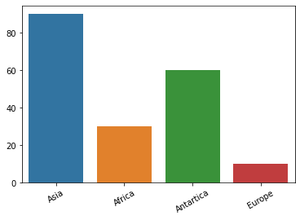Rotating Y-axis Labels in Matplotlib

We use plt.xticks(rotation=#) where # can be any angle by which we want to rotate the y labels

## Python3

 `import` `numpy as np``import` `matplotlib.pyplot as plt`` ` `data ``=` `{``'Cristopher'``: ``20``, ``'Agara'``: ``15``, ``'Jayson'``: ``30``,``        ``'Peter'``: ``35``}``courses ``=` `list``(data.keys())``values ``=` `list``(data.values())`` ` `fig ``=` `plt.figure(figsize``=``(``8``, ``5``))`` ` `# creating the bar plot``plt.bar(courses, values, color``=``'blue'``, width``=``0.4``)``plt.yticks(rotation``=``45``)``plt.xlabel(``"Names"``)``plt.ylabel(``"Age of the person"``)``plt.show()`

Output: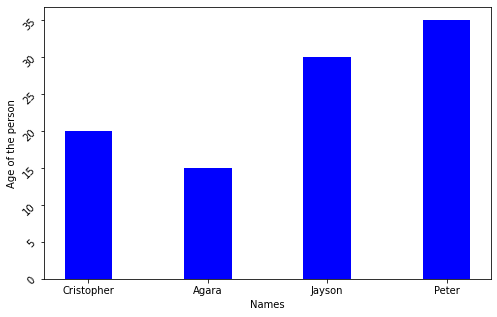Rotating Y-axis Labels in Seaborn

By using FacetGrid we assign barplot to variable ‘g’ and then we call the function set_yticklabels(labels=#the scale we want for y label, rotation=*) where * can be any angle by which we want to rotate the y labels

## Python3

 `import` `seaborn as sns``import` `matplotlib.pyplot as plt`` ` `g ``=` `sns.barplot(x``=``[``"Asia"``, ``"Africa"``, ``"Antartica"``, ``"Europe"``],``                ``y``=``[``90``, ``30``, ``60``, ``10``])`` ` `g.set_yticklabels(labels``=``[``0``, ``20``, ``40``, ``60``, ``80``], rotation``=``30``)`` ` `# Show the plot``plt.show()`

Output: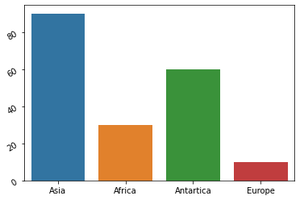Attention geek! Strengthen your foundations with the Python Programming Foundation Course and learn the basics.

To begin with, your interview preparations Enhance your Data Structures concepts with the Python DS Course.

My Personal Notes arrow_drop_up